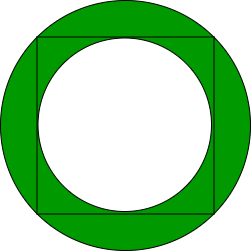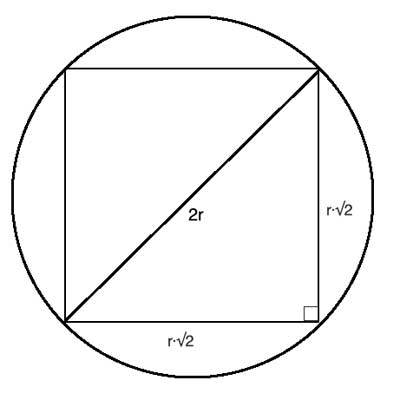Related Articles

# Area of the circle that has a square and a circle inscribed in it

• Last Updated : 09 Apr, 2021

Given the side of a square a which is kept inside a circle. It keeps expanding until all four of its vertices touch the circumference of the circle. Another smaller circle is kept inside the square now and it keeps expanding until its circumference touches all the four sides of the square. The outer and the inner circle form a ring. Find the area of this shaded part as shown in the image below.Examples:

Input: a = 3
Output: 7.06858
Input: a = 4
Output: 12.566371

Approach:From the above figure, R = a / sqrt(2) can be derived where a is the side length of the square. The area of the outer circle is (pi * R * R).Let s1 be the area of the outer circle (pi * R * R) and s2 be the area of the inner circle (pi * r * r). Then the area of the ring is s1 – s2.
Below is the implementation of the above approach:

## C++

 `// C++ implementation of the approach``#include ``using` `namespace` `std;` `// Function to return the required area``float` `getArea(``int` `a)``{` `    ``// Calculate the area``    ``float` `area = (M_PI * a * a) / 4.0;``    ``return` `area;``}` `// Driver code``int` `main()``{``    ``int` `a = 3;` `    ``cout << getArea(a);` `    ``return` `0;``}`

## Java

 `// Java implementation of the approach``class` `GFG {` `    ``// Function to return the required area``    ``static` `float` `getArea(``int` `a)``    ``{` `        ``// Calculate the area``        ``float` `area = (``float``)(Math.PI * a * a) / ``4``;``        ``return` `area;``    ``}` `    ``// Driver code``    ``public` `static` `void` `main(String args[])``    ``{``        ``int` `a = ``3``;``        ``System.out.println(getArea(a));``    ``}``}`

## Python3

 `# Python3 implementation of the approach``import` `math` `# Function to return the required area``def` `getArea(a):``    ` `    ``# Calculate the area``    ``area ``=` `(math.pi ``*` `a ``*` `a) ``/` `4``    ``return` `area``    ` `# Driver code``a ``=` `3``print``(``'{0:.6f}'``.``format``(getArea(a)))`

## C#

 `// C# implementation of the approach``using` `System;` `class` `GFG``{` `    ``// Function to return the required area``    ``static` `float` `getArea(``int` `a)``    ``{` `        ``// Calculate the area``        ``float` `area = (``float``)(Math.PI * a * a) / 4;``        ``return` `area;``    ``}` `    ``// Driver code``    ``public` `static` `void` `Main()``    ``{``        ``int` `a = 3;``        ``Console.Write(getArea(a));``    ``}``}` `// This code is contributed by mohit kumar 29`

## Javascript

 ``
Output:
`7.06858`

Attention reader! Don’t stop learning now. Participate in the Scholorship Test for First-Step-to-DSA Course for Class 9 to 12 students.

My Personal Notes arrow_drop_up